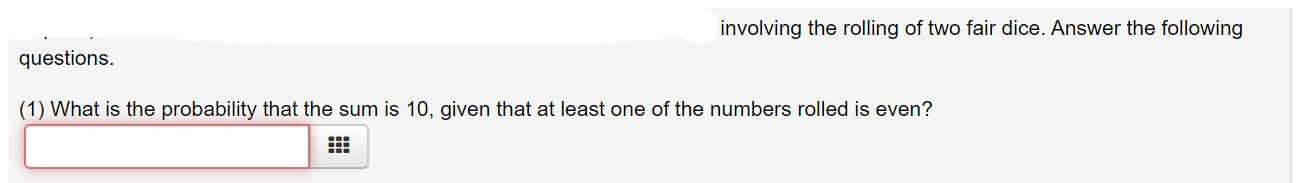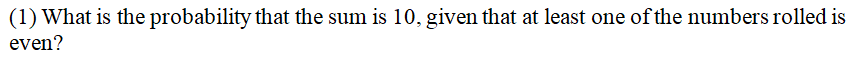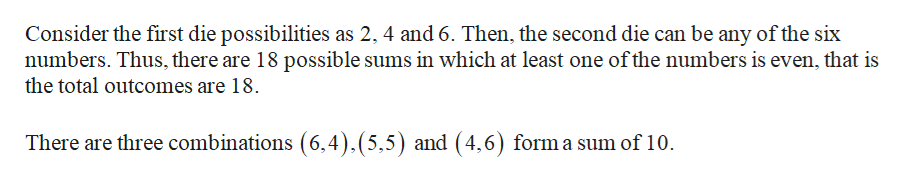# involving the rolling of two fair dice. Answer the followingquestions.(1) What is the probability that the sum is 10, given that at least one of the numbers rolled is even?

Question
2 viewshelp_outlineImage Transcriptioncloseinvolving the rolling of two fair dice. Answer the following questions. (1) What is the probability that the sum is 10, given that at least one of the numbers rolled is even? fullscreen
check_circle

Step 1...help_outlineImage TranscriptioncloseConsider the first die possibilities as 2, 4 and 6. Then, the second die can be any of the six numbers. Thus, there are 18 possible sums in which at least one of the numbers is even, that is the total outcomes are 18. There are three combinations (6,4),(5,5) and (4,6) form a sum of 10. fullscreen

### Want to see the full answer?

See Solution

#### Want to see this answer and more?

Solutions are written by subject experts who are available 24/7. Questions are typically answered within 1 hour.*

See Solution
*Response times may vary by subject and question.
Tagged in

### Math